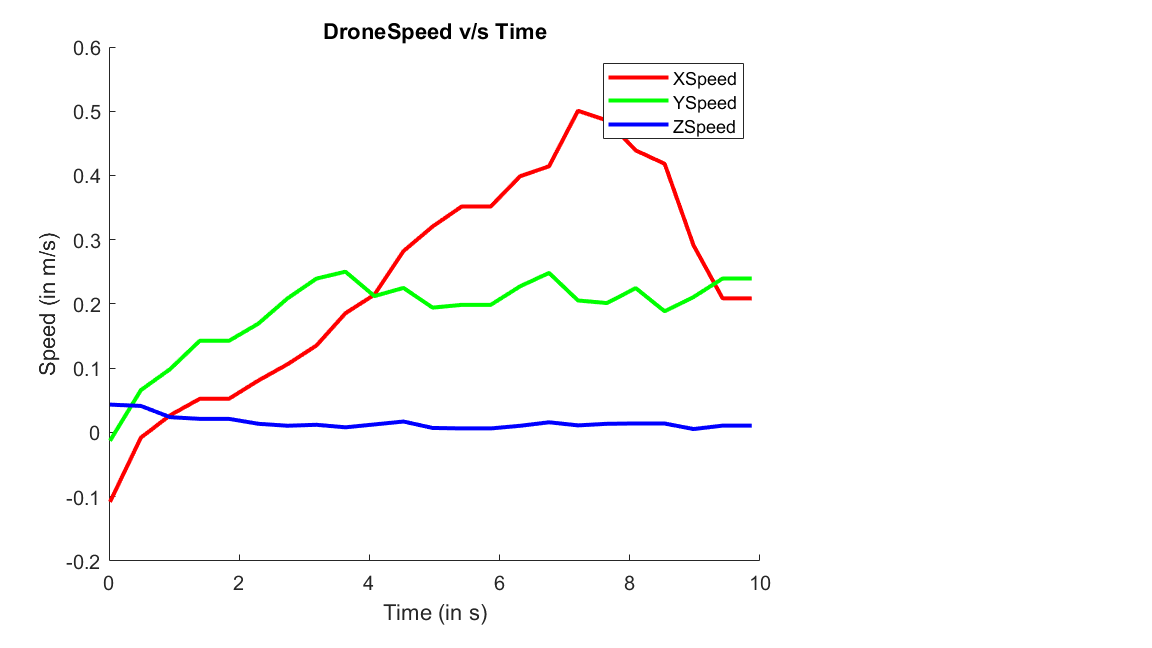# Read and Plot Navigation data Using MATLAB Support Package for Parrot Drones

This example shows how to use the MATLAB® Support Package for Parrot® drone to acquire and plot real-time navigation data of the Parrot drone.

### Introduction

The MATLAB Support Package for Parrot Drones enables you to control and read the in flight navigation data of the drone.

In this example, you will learn to read navigation data of the Parrot drone such as the speed, orientation, and height using MATLAB commands.

### Required Hardware

To run this example you need the following:

• A fully charged Parrot FPV drone

• A computer with a WiFi connection

### Task 1 — Hardware setup

• Power on the Parrot FPV drone.

• Connect your computer to the drone's Wifi network.

### Task 2 — Create a parrot object

Create a `parrot` object.

` p = parrot();`

### Task 3 — Take-off the drone

Start the Parrot FPV drone flight from a level surface.

Execute the following command at the MATLAB command prompt the takeoff of the drone.

` takeoff(p);`

### Task 3 — Initialize MATLAB `animatedline` and `figure` window properties

This task shows you how to initialize MATLAB to plot the navigation data.

Use MATLAB `animatedline` to plot the variation in speed along the X, Y, and Z axes, separately.

Initialize the figure handle and create animated line instances hx, hy, and hz corresponding to speeds along the X, Y, and Z axes, respectively.

``` f = figure; hx = animatedline('Color', 'r', 'LineWidth', 2); hy = animatedline('Color', 'g', 'LineWidth', 2); hz = animatedline('Color', 'b', 'LineWidth', 2); title('DroneSpeed v/s Time'); xlabel('Time (in s)'); ylabel('Speed (in m/s)'); legend('XSpeed', 'YSpeed', 'ZSpeed');```

Keep flying the drone along the desired path (forward diagonal path in this example) for 10 seconds and plot navigation data (speed) during this flight.

The default value of `duration` in `move` function is 0.5 seconds.

``` flightTime = 10; tObj = tic; while(p.BatteryLevel > 10 && toc(tObj) < flightTime) move(p, 'Pitch', deg2rad(-4), 'Roll', deg2rad(4)); speed = readSpeed(p); tStamp = toc(tObj); addpoints(hx, tStamp, speed(1)); addpoints(hy, tStamp, speed(2)); addpoints(hz, tStamp, speed(3)); drawnow; pause(0.1); end```### Task 5 — Land the drone

Land the drone.

```land(p); ```

### Task 6 — Clean up

When finished, clear the connection to the Parrot drone.

```clear p; ```